# Alkanes

### Alkanes

#### Lessons

In this lesson, we will learn:
• The definition of an alkane, their general formula and the major types of them.
• The major properties of alkanes and differences to other hydrocarbons.
• How to name branched, unbranched and cyclic alkanes and draw their structural formula.

Notes:

• Alkanes are the simplest hydrocarbons. The definition of an alkane is a saturated hydrocarbon with only single bonds between carbon atoms.

• Alkanes are a homologous series in organic chemistry.
• A homologous series is a set of compounds with the same general formula, with each compound differing from the next by CH2.
• Other examples of a homologous series are alkenes (general formula C$_n$H$_{2n}$), alkynes (C$_n$H$_{2n-2}$) and alcohols (C$_n$H$_{2n+2}$O).

• Alkanes have the general formula: C$_n$H$_{2n+2}$. This means that in an alkane, the number of hydrogen atoms is double the number of carbon atoms plus two.
• This reflects that every carbon atom in the chain is bonded to two carbon atoms (one before and one after it in the chain) and two hydrogen atoms each.
• This is except for the carbons on the beginning and end of the chain – those are bonded to three hydrogens each.

• Alkanes are unreactive compared to other hydrocarbons because they have no double or triple bonds – for them to react requires breaking a strong C-C bond which is difficult.

• Most alkanes are insoluble in water – they don't mix! They are more lipophilic (fat loving) than hydrophilic, so they are more easily dissolved in oils and fats instead of water.

• Hydrocarbons are flammable – methane, the simplest hydrocarbon, is also known as natural gas, and all of the shorter chain alkanes are volatile, flammable gases or liquids. Many of them are used as fuels!

• Alkanes will react with oxygen in a combustion reaction to produce carbon dioxide and water. For example, the reaction of methane and oxygen is given below:
• CH4 + 2O2CO2 + 2H2O

• Straight chain alkanes are known as 'unbranched' alkanes because the molecule is simply a straight chain of carbon atoms – each carbon is only bonded to two other carbon atoms in the middle of the chain, while the two chain ends are only bonded to one. They are named using the simple rules we saw in the "introduction to organic chemistry" lesson. See the table and examples below. Notice they all fit the alkane general formula: C$_n$H$_{2n+2}$
•  Length of main chain Root name Alkane name Molecular formula 1 Meth- Methane CH4 2 Eth- Ethane C2H6 3 Prop- Propane C3H8 4 But- Butane C4H10 5 Pent- Pentane C5H12 6 Hex- Hexane C6H14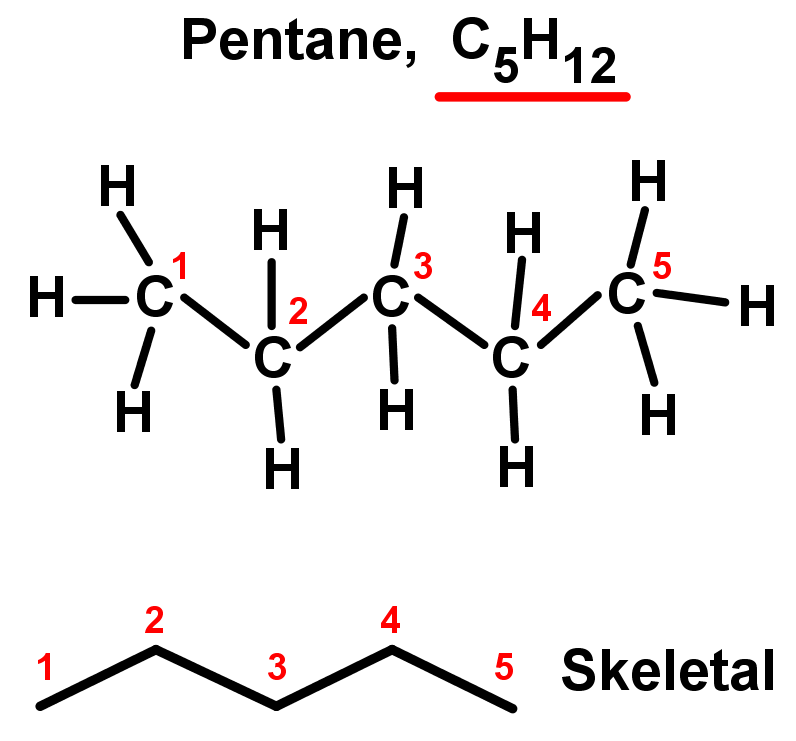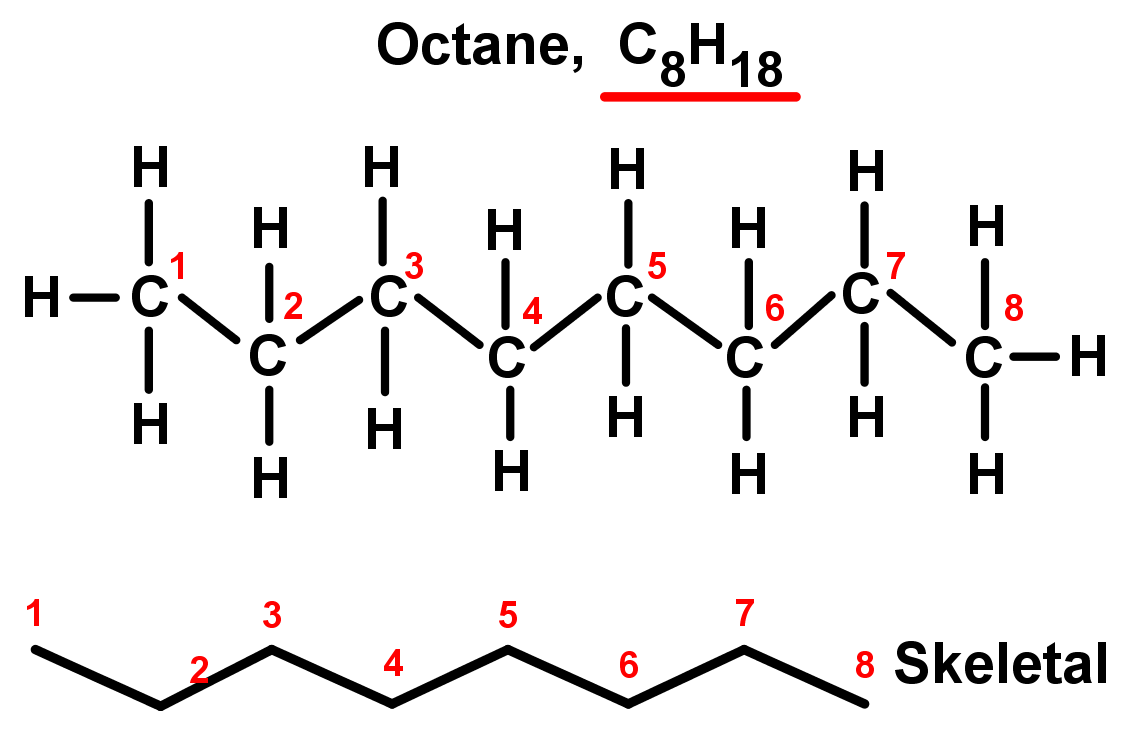• Branched alkanes or substituted alkanes contain carbon atoms in the middle of the chain bonded to carbon atoms that branch off of the main chain. This can create more than one possible 'chain lengths' – the longest chain is always considered the main chain, and the shorter ones are considered only branches. Naming branches uses the root words for the main chain, but ending in –yl to show it is a branch.
• Note one H atom is missing from the alkyl branch formulae. This because what would have been a C-H bond is instead a bond to the main carbon chain. See the table below:
• You must also add a number before this prefix, to show which carbon atom in the main chain the branch is found on. The numbering should always be the lowest possible.
 Chain length of branch Alkyl root Formula of alkyl branch 1 Methyl- -CH3 2 Ethyl- -C2H5 3 Propyl- -C3H7 4 Butyl- -C4H9 5 Pentyl- -C5H11 6 Hexyl- -C6H13

• Using these rules we will look at two examples of hydrocarbons.
• In the first example, there are two branches of one carbon each – they are equal so it doesn't matter which you choose; one can be the main chain and the other the branch. So the longest chain is 5 carbons, with a single branch of 1 carbon. The 1 carbon branch is on the second carbon in the chain, because we always number using the lowest values possible (it could be on the 4th carbon if we counted the other way). So here have:
• A branch on the second carbon in the main chain: 2-
• A branch of 1 carbon length: methyl-
• A 5 carbon main chain alkane: pentane
• Combining these we have a compound named 2-methylpentane.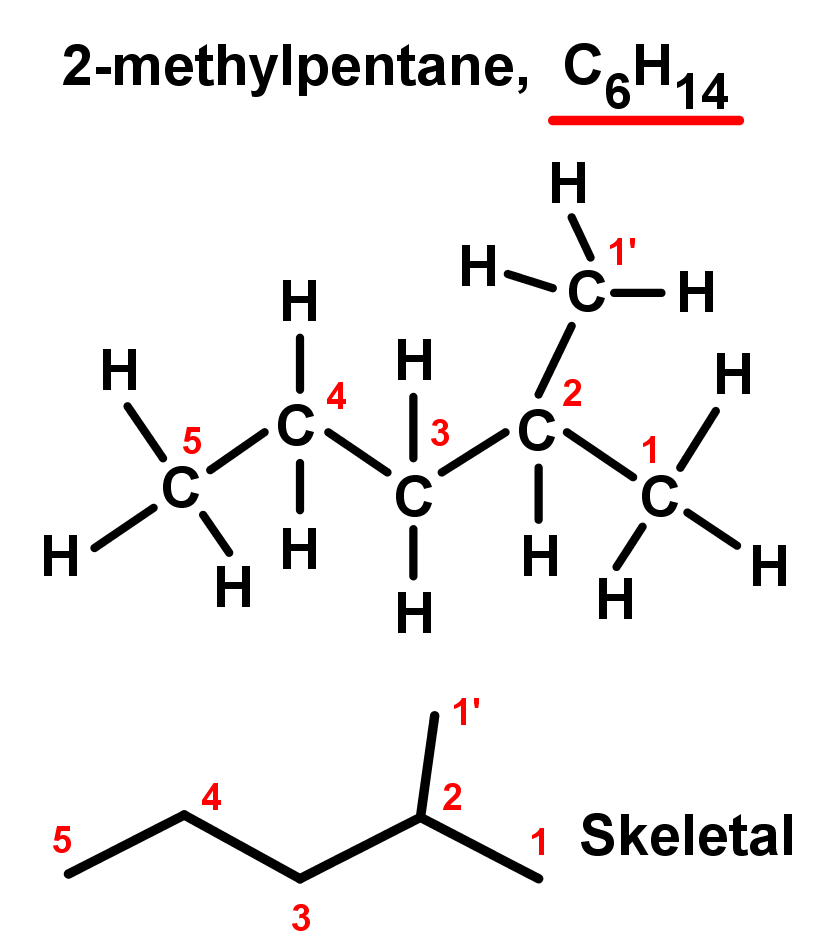• In the second example, there are two end branches of two carbons each – again they are equal so we can pick one as the main chain and the other can be considered a branch. Again, number the branch off of the main chain with the lowest value possible, we will choose 3 instead of 4. We now have:
• A branch on the third carbon in the main chain: 3-
• A branch of 2 carbon atoms in length: ethyl-
• A 6 carbon main chain alkane: hexane
• Combining these, we have a compound named 3-ethylhexane.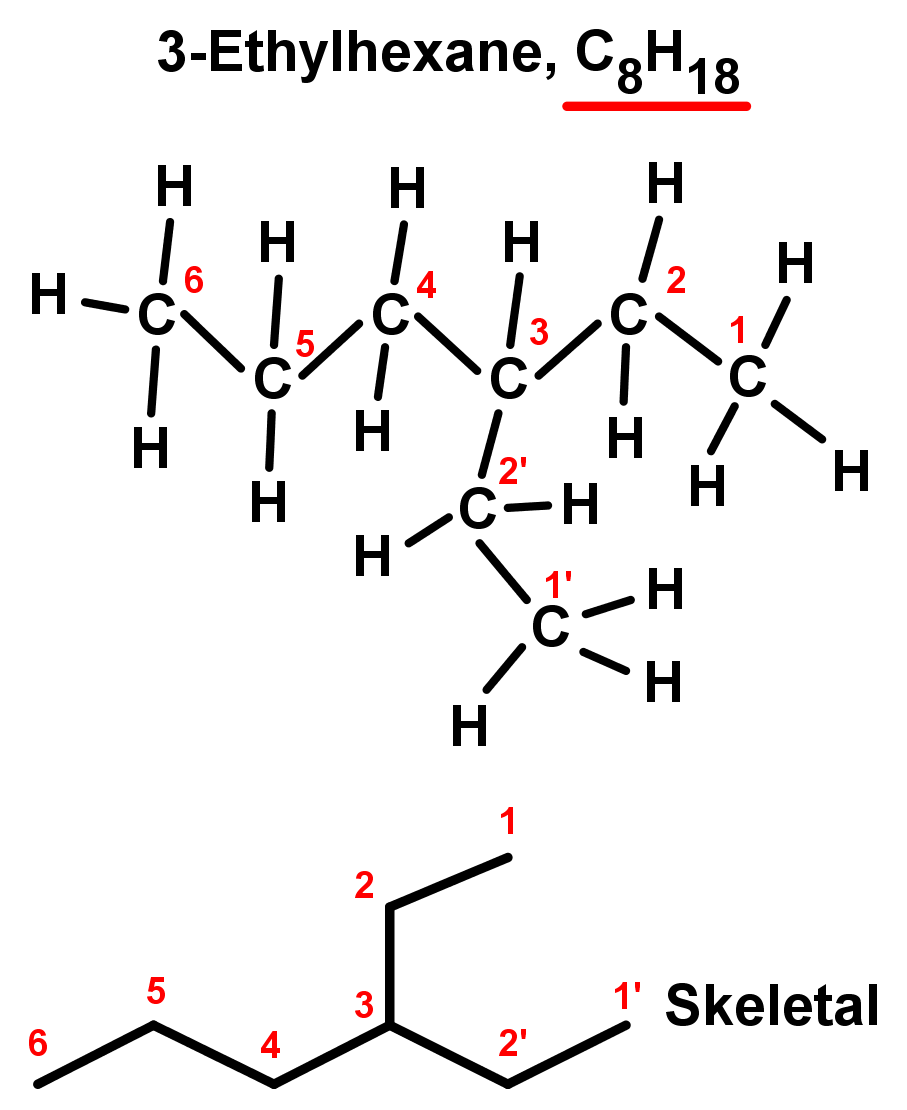• If branched alkanes have more than one type of branch, branches must be named in alphabetical order. For example, 'e' comes before 'm' in the alphabet, so ethyl chains should be named before methyl chains. See the example below:

•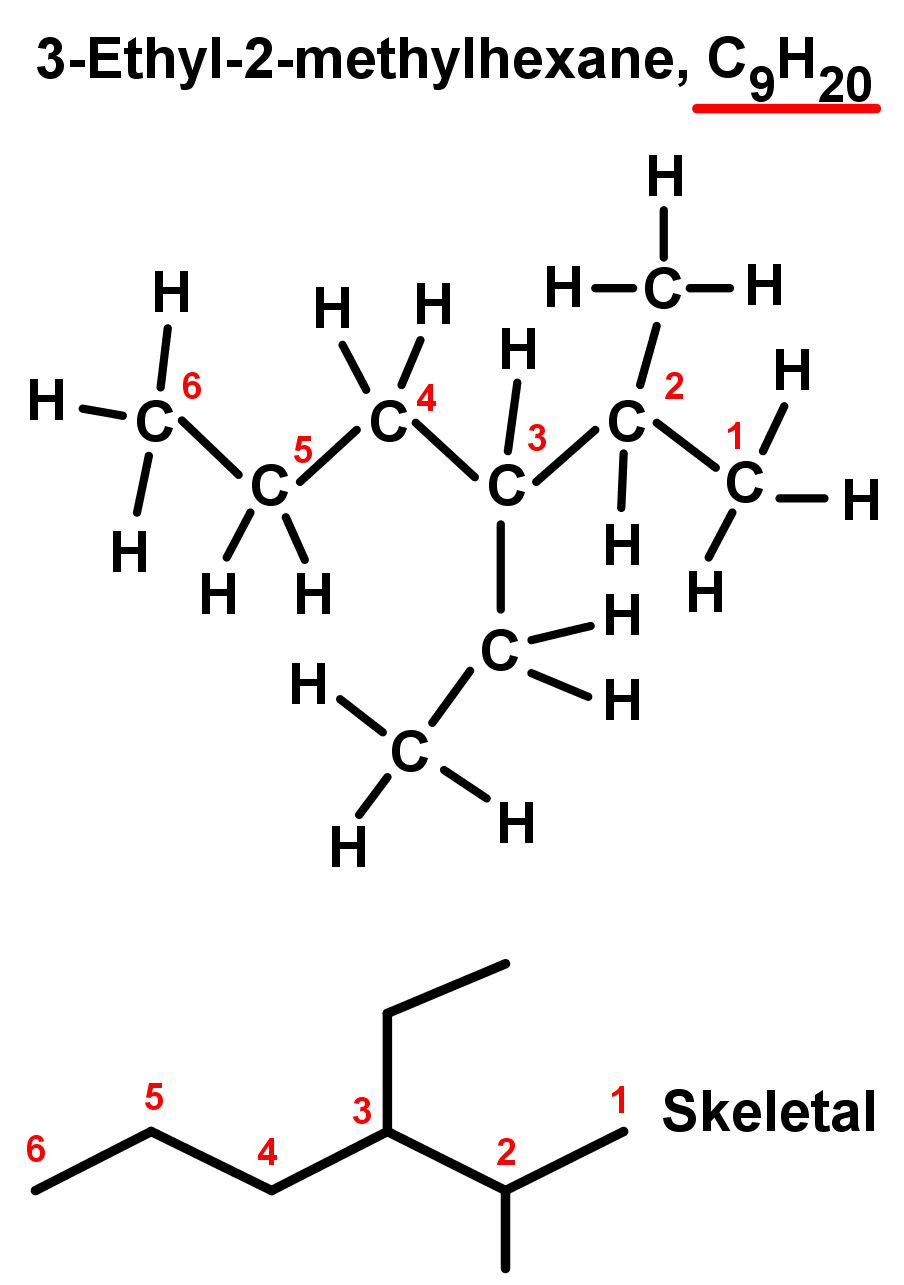• If you have an organic compound with more than one of the same type of branch or substituent, you need to say how many of these branches there are, and at which carbons in the chain they're found. This is done systematically:
• Two of the same substituents: di-
• Three of the same substituents: tri-
• Four: tetra-
• Five: penta-
• Six: hexa-
See the example below: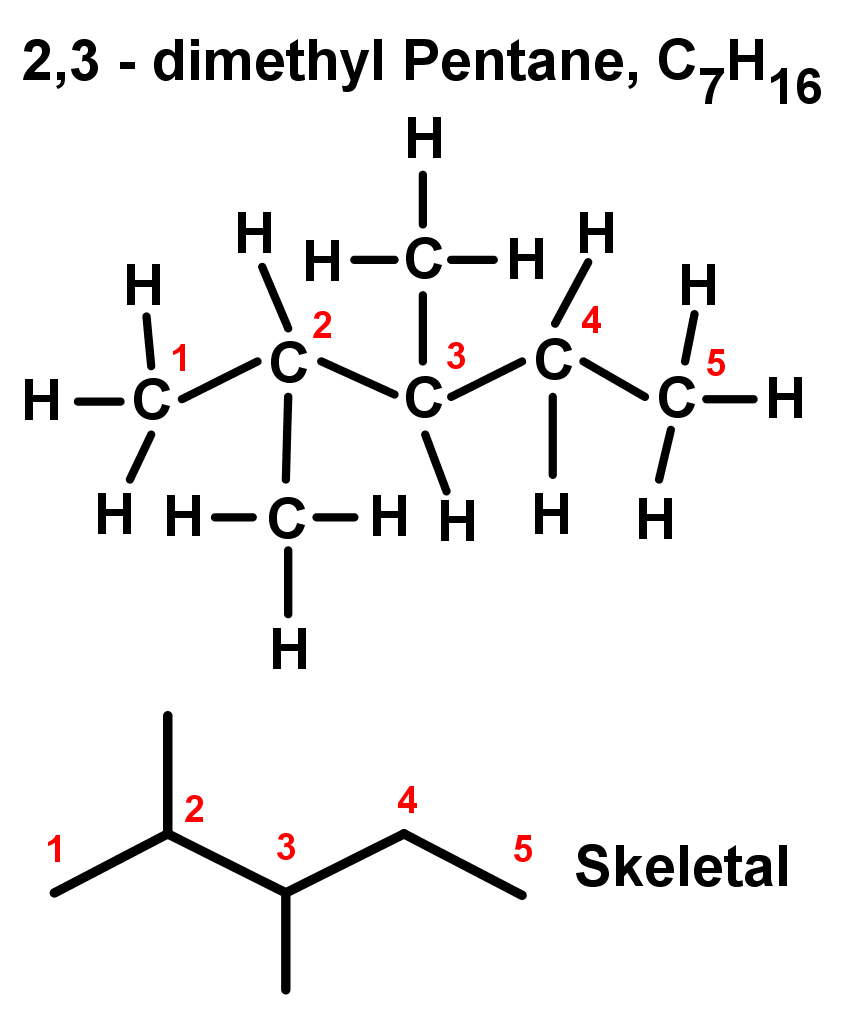• Some hydrocarbons have the beginning and end of the chain bonded together, making a closed cyclic ring. These are unique chemical compounds with different properties to the open chain of the same carbon length. Because the ends of the chain are bonded together, cyclic alkanes have a different general formula to the rest of the alkanes. The general formula for cyclic alkanes is C$_n$H$_{2n}$, like an alkene.
• To name cyclic compounds, the same basic rule of 'chain' length applies, with slight changes:
• Unbranched rings: Find the number of carbon atoms in the ring. This would be your original chain length. Then add 'cyclo-' in front. See the example: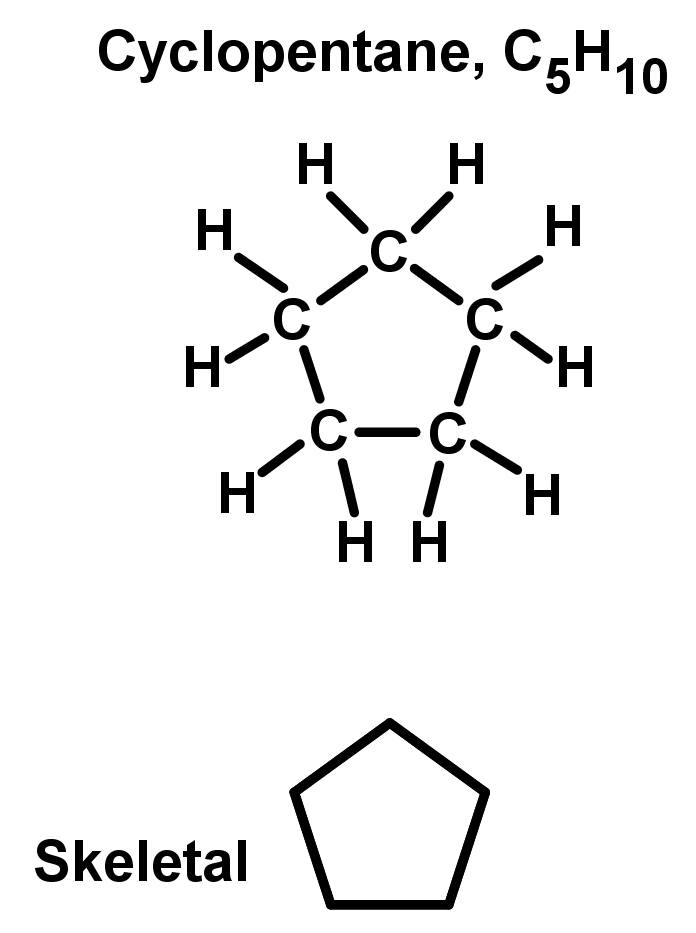• Rings with one branch or substituent: Start numbering from the ring carbon bonded to the branch. Because the ring has no 'start' or 'end', you can just pick the carbon bonded to the branch as number 1. Because doing this is implied, we don't need to say 1-ethylcyclooctane, just ethylcyclooctane (see the example below).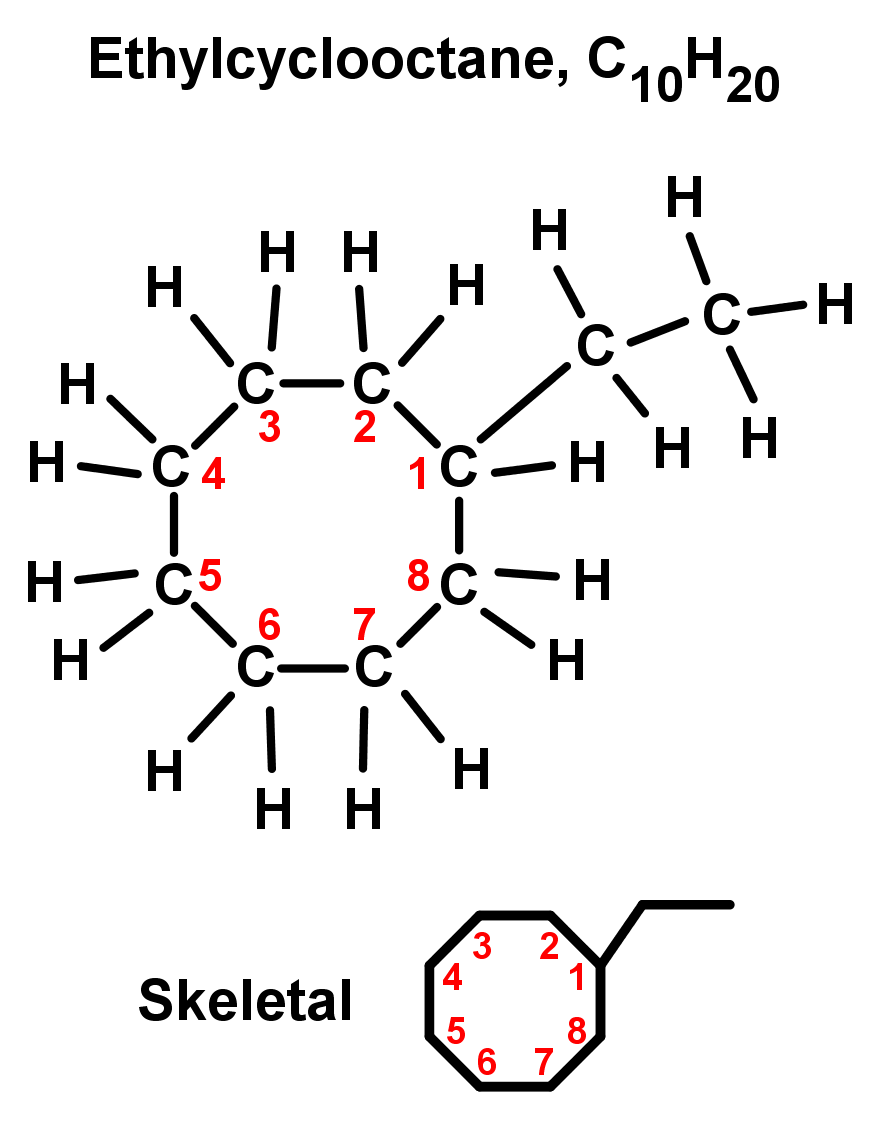• Rings with more than one branch or substituent: Start numbering at the substituent that comes first in A-Z order, and count from there following the lowest number rule. See the example below: ethyl comes before methyl in A-Z order, therefore it is named and numbered first. The rest of the ring is then numbered from here to give the lowest numbering possible (so '1-ethyl-3-methyl…' instead of going the other way around, which would give '1-ethyl-5-methyl…').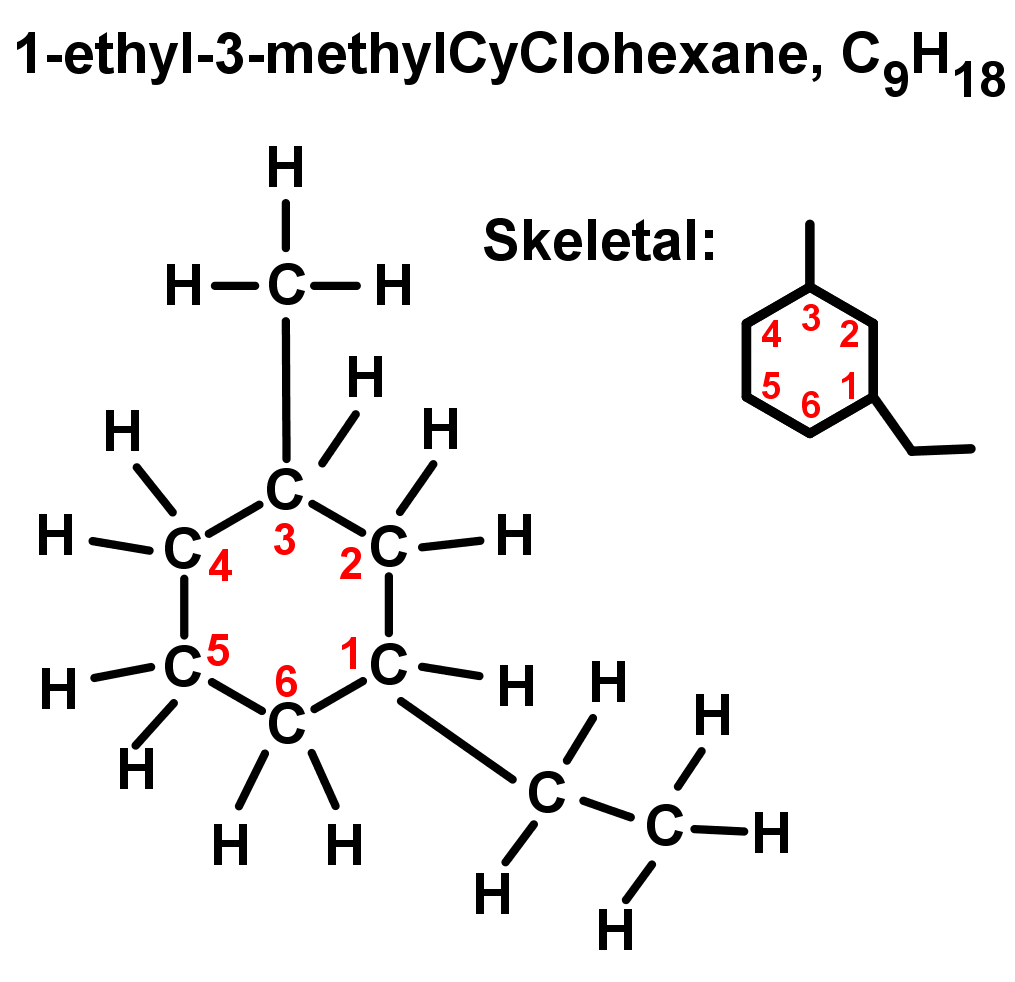• Notice that these branched alkanes and cycloalkanes can have the same molecular formula as some unbranched, straight chain alkanes and alkenes. These are examples of isomers – where the molecular formulae are the same but the structural formulae are different. This is very important as they are not the same chemical, they are unique and have different properties. This is another major reason why we use structural or skeletal formula when describing chemicals.
• Introduction
Alkanes - Introduction
a)
What is an alkane?

b)
Alkane general formula and examples.

c)
Reactions and properties of alkanes.

d)
Unbranched alkanes.

e)
Branched alkanes.

f)
Cyclic alkanes.

g)
How can different alkanes have the same chemical formula?

• 1.
Recall the difference between alkanes and other hydrocarbons (alkenes and alkynes).
Look at the following molecular formulae and identify which formulae show alkanes.
i) C$_2$H$_4$
ii) C$_4$H$_{10}$
iii) C$_3$H$_6$
iv) C$_{10}$H$_{22}$
v) CH$_4$
vi) C$_2$H$_2$

• 2.
Apply the rules of organic nomenclature to branched and unbranched alkanes.
From the descriptions, suggest the IUPAC systematic name of the following compounds:
a)
An alkane with 6 carbons in the main chain, and a 1 carbon branch at the 3rd carbon.

b)
An alkane with 4 carbons in the main chain, and a 1 carbon branch at the 2nd carbon.

c)
An alkane with 8 carbons in the main chain, and a 2 carbon branch at the 3rd carbon.

d)
An alkane with 5 carbons in the main chain with the two end carbons bonded together.

• 3.
Draw the structural formula of simple branched, unbranched and cyclic alkanes.
Use the IUPAC systematic names of the compounds below to draw their structural formula.
a)
2-methylhexane.

b)
Heptane.

c)
Methylpropane.

d)
3-ethyloctane.

e)
Methylcyclohexane.

f)
1-ethyl-2-methylcyclohexane.